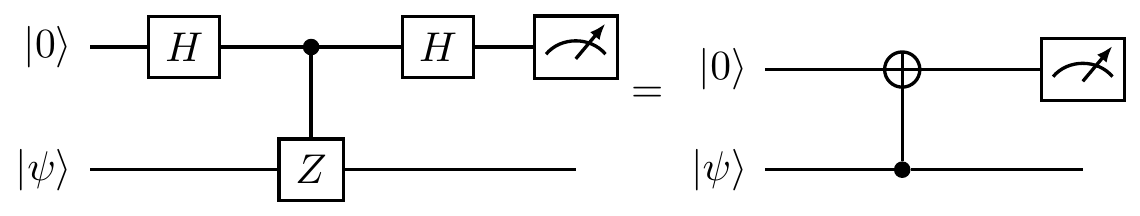## 9.3 Random isometries

In many applications, including quantum communication and quantum error correction, it is useful to encode a quantum state of one system into a quantum state of a larger system. Such operations are described by isometries.170 You may think about isometries as a generalisation of unitaries: like unitaries, they preserve inner products; unlike unitaries, they are maps between spaces of different dimensions.

Let \mathcal{H} and \mathcal{H}' be Hilbert spaces such that \dim\mathcal{H}\leqslant\dim\mathcal{H}'. An isometry is a linear map V\colon\mathcal{H}\to\mathcal{H}' such that V^\dagger V=\mathbf{1}_{\mathcal{H}}

Isometries preserve inner products, and therefore also the norm and the metric induced by the norm.

An isometry V\colon\mathcal{H}\to\mathcal{H}' maps the whole Hilbert space \mathcal{H} onto a subspace of \mathcal{H}'. As a consequence, the matrix representation of an isometry is a rectangular matrix formed by selecting only a few of the columns from a unitary matrix. For example, given a unitary U we can construct an isometry V as follows:The fact that an isometry V preserves the inner products comes from the fact that we require V^\dagger V=\mathbf{1}_{\mathcal{H}}; we do not require VV^\dagger=\mathbf{1}_{\mathcal{H'}}. Indeed, if we required both of these, then that would be equivalent to asking for V to be unitary. The operator VV^\dagger is a projector operator acting on \mathcal{H}', which projects onto the image of \mathcal{H} under the isometry V, as we can see by expressing V in Dirac notation: V = \sum_i |b_i\rangle\langle a_i|, where the |a_i\rangle form an orthonormal basis in \mathcal{H}, and the |b_i\rangle are just orthonormal (but not necessarily spanning) vectors in \mathcal{H}'; in the special case where V is unitary, the orthonormal vectors |b_i\rangle form an orthonormal basis in \mathcal{H}'. Writing V in this form, it is clear that V^\dagger V=\sum_i |a_i\rangle\langle a_i|=\mathbf{1}, and that VV^\dagger = \sum_i |b_i\rangle\langle b_i| projects on the subspace spanned by |b_i\rangle.

Although isometries are strictly more general than unitaries, an fundamentally important fact is that isometries still represent physically admissible operations: they can be implemented by bringing two systems together (via tensoring) and then applying unitary transformations to the composite system. That is, take some system \mathcal{A} in state |\psi\rangle, and bring in another system \mathcal{B} in some fixed state |b\rangle; applying some unitary U to the combined system \mathcal{A}\mathcal{B} then gives an isometry from \mathcal{H}=\mathcal{H}_\mathcal{A} to \mathcal{H}'=\mathcal{H}_\mathcal{A}\otimes\mathcal{H}_\mathcal{B}, i.e. the result is a linear map V defined by V\colon |\psi\rangle \longmapsto |\psi\rangle|b\rangle \longmapsto U(|\psi\rangle|b\rangle).

Any isometry is a quantum channel, since any quantum state described by the state vector |\psi\rangle (or by a density operator \rho) is transformed as |\psi\rangle\longmapsto V|\psi\rangle (or as \rho\mapsto V\rho V^\dagger), and the normalisation condition is exactly the defining property of isometries: V^\dagger V =\mathbf{1}.

Isometries are incredibly important when it comes to error correction, and we will see them again much more in Section 13.2.

1. The word isometric (like pretty much most of the fancy words you come across in this course) comes from Greek, meaning “of the same measures”: isos means “equal”, and metron means “a measure”, and so an “isometry” is a transformation that preserves distances.↩︎